Courses

# Sample Question Paper - 9 Class 9 Notes | EduRev

## Class 9 : Sample Question Paper - 9 Class 9 Notes | EduRev

The document Sample Question Paper - 9 Class 9 Notes | EduRev is a part of the Class 9 Course Sample Papers For Class 9.
All you need of Class 9 at this link: Class 9

SECTION - A

Q.1. Which of the figure represents uniform linear motion?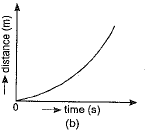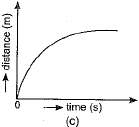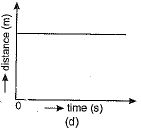Ans. (a) The graph shown in Fig. (a) represents uniform linear motion because in this case displacement-time graph is a straight line inclined to the time axis.

Q.2. State any one difference between pure and impure substances.
Ans.
Pure substance contains only one kind of particles or molecules while impure substance contains more than one kind of particles or molecules.

Q.3. Which of the following are true for an element?
(i) Atomic number = number of protons + number of electrons
(ii) Mass number = number of protons + number of neutrons
(iii) Atomic number = number of protons = number of neutrons
(iv) Atomic number = number of protons = number of electrons
(a) (i) and (ii)
(b) (i) and (iii)
(c) (ii) and (iii)
(d) (ii) and (iv)
Or
Which of the following in figure do not represent Bohr's model of an atom correctly?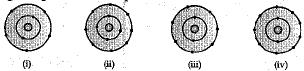(a) (i) and (ii)
(b) (ii) and (iii)
(c) (ii) and (iv)
(d) (i) and (iv)
Ans.
(d) (ii) and (iv)
Atomic number = Number of protons = Number of electrons in neutral atom
Mass number = Number of protons + Number of neutrons
or
(c) (ii) and (iv)
Figures (ii) and (iv) do not correctly represent the Bohr's model of an atom. It is because maximum number of electrons in K(I) shell is 2, not 4, so (ii) is wrong and maximum capacity of L(II) shell is 8 electrons, not 9. So, (iv) is also wrong.

Q.4. An astronaut can’t hear his companion at the surface of moon because
(a) produced frequencies are above the audible frequencies.
(b) there is no medium for sound propagation.
(c) temperature is too low.
(d) there are too many craters on the surface of moon.
Ans.
(b) An astronaut cannot hear his companion on the surface of moon, as he does on earth. It is because there is no atmosphere on the surface of moon. In the absence of a medium sound wave cannot be propagated.

Q.5. A car travels 100 km east and then 100 km south. Finally, it comes back to the starting point by the south-east route. Throughout the journey the speed is constant at 60 km h-1. The average velocity for the whole journey if time taken is 3.3 hours is:
(a) 60 km h-1

(b) 90 km h-1
(c) 0 km h-1
(d) 180 km h-1
Or
The displacement of a body is proportional to the cube of the time lapsed. The magnitude of the acceleration is:
(a) increasing with time

(b) decreasing with time
(c) constant
(d) zero
Ans.
(c) or (a)

Q.6. Two chemical species X and Y combine together to form a product P which contains both X and Y, X + Y → P, X and Y cannot be broken doiwn into simpler substances by simple chemical reactions. Which of the following concerning the species X, Y and P are correct?
(i) P is a compound
(ii) X and Y are compounds
(iii) X and Y are elements
(iv) P has a fixed composition
(a) (i), (ii) and (iii)
(b) (i), (ii) and (iv)
(c) (ii), (iii) and (iv)
(d) (i), (iii) and (iv)
Ans.
(i), (iii) and (iv)
In this reaction, X and Y cannot be broken down into simpler substances by chemical reactions. Therefore, X and Y are elements. A compound is a substance made up of two or more elements chemically combined in a fixed proportion by mass. Therefore, P is a compound, having a fixed composition.

Q.7. Symbols of silicon and uranium respectively are
(a) SI and Ur
(b) SI and Un
(c) Si and U
(d) Si and Ur
Ans.
(c) Si and U

Q.8. If you live in a overcrowded and poorly ventilated house, it is possible that you may suffer from which of the following diseases:
(a) Cancer
(b) AIDS
(c) Air-borne diseases
(d) Cholera
OR
The term "water pollution" can be defined in several ways. Which of the following statements does not give the correct definition?
(a) The addition of undesirable substances to water bodies
(b) The removal of desirable substances from water bodies
(c) A change in pressure of the water bodies
(d) A change in temperature of the water bodies
Ans.
(c) or (c)

Q.9. Weeds affect the crop plants by
(a) Killing of plants in field before they grow
(b) Dominating the plants to grow
(c) Competing for various resources of crops (plants) causing low availability of nutrients
(d) All of the above
Ans.
(d) All of the above
Weeds are unwanted plants in the cultivated fields. They compete with main crop pants for nutrients and reduce the growth of crops in manu ways.

Q.10. The nitrogen molecule present in air can be converted into nitrates and nitrites by
(а) a biological process of nitrogen fixing bacteria present in soil.
(b) a biological process of carbon fixing factor present in the soil.
(c) any of the industries manufacturing nitrogenous compounds.
(d) the plants used as cereal crops in field.
Ans.
(a) Nitrogen fixing bacteria present in the nodules of leguminous plants convert molecular nitrogen into nitrites and nitrates.

Q.11. Tincture of iodine has antiseptic properties. This solution is made by dissolving:
(a) Iodine in potassium iodide
(b) Iodine in vaseline
(c) Iodine in water
(d) Iodine in alcohol
Ans.
(d)

Q.12. Give reason, why a block of plastic when released under water comes up to the surface of water.
Ans.
Density of a plastic block is less than the density of water, and hence, the upthrust buoyant force of water on plastic block is more and it floats on the water surface.

Q.13. Sound waves can travel through any medium solid, liquid and gas as well as vaccum.
Ans. False - Sound waves cannot travel through vacuum.

Q.14. Assertion: When the displacement of a body is directly proportional to the square of the time. Then the body is moving with uniform acceleration.
Reason: The slope of velocity-time graph with time axis gives acceleration.
(i) A (ii) B (iii) C (iv) D
Ans.
(ii)

SECTION - B

Q.15. Draw velocity-time graphs for the following cases:
(a) When the object is in uniform motion.
(b) When the object is in uniformly accelerated motion with initial velocity (i) zero (ii) non-zero.
(c) When the object has uniformly retarded velocity.
Ans.
(a) An object in uniform motion has a constant velocity and its velocity - time graph is a straight line parallel to the time axis as shown in figure (a).
(b) (i) When the object is in uniformly accelerated motion with initial velocity 'u' to be zero, the velocity - time graph is a straight line passing through the origin and having a positive slope as shown in figure (b).
(ii) If u is finite and motion is uniformly accelerated one then the velocity-time graph of the object is as shown in figure (c).
(c) The velocity-time graph for uniformly retarded motion is shown in figure (d) the graph is a straight line whose slope is negative.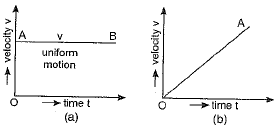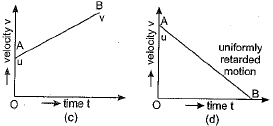Q.16. To make a saturated solution, 36 g of sodium chloride is dissolved in 100g of water at 293 K. Find its concentration at this temperature.
OR
How will you separate a mixture containing kerosene and petrol (difference in their boiling points is more than 25°C), which are miscible with each other?
Ans. Mass of sodium chloride (Solute) = 36 g
Mass of water (Solvent) = 100 g
Mass of solution (Solvent + Sulute) = 100 + 36 = 136 g
Concentration of sodium chloride solution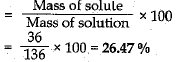OR
To separate a mixture containing kerosene and petrol which are miscible with each other, fractional distillation process is employed.
1. Take a mixture of kerosene and petrol in a distillation flask and heat it on a low flame.
2. The petrol has lower boiling point than kerosene. It vaporises early as compared to kerosene. When the vapours of petrol and kerosene are passed into fractionating column, the kerosene in the form of liquid (after condensation) flows back in the distillation flask.
3. When the temperature starts rising, the receiver is replaced by another receiver to collect the kerosene.
4. The vapours of petrol pass into Liebig's condenser, these vapours get liquefied and flow into receiver.

Q.17. The kinetic energy of an object moving with a velocity of 5 m/s is 25 J. Find the mass of the object. What will be its kinetic energy when its velocity is made : (i) two times, (ii) three times? Justify your answers.
OR
A boy is pulling a cart by supplying a constant force of 8 N on a straight path of 20 m. On a round about of 10 m diameter he forgets the path and takes Vfz turns and then continues on the straight path for another 20 m. Find the net workdone by the boy on the cart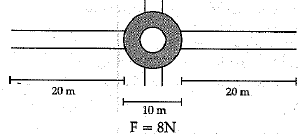Ans.
Mass = m,v = 5 m/s, K.E. = 25 J
K.E. = 1/2 mv2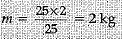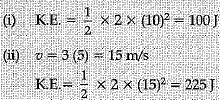OR
Work done, W= F x s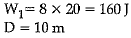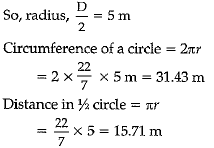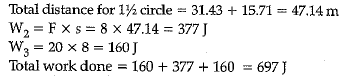Q.18. State the SI unit of temperature. Mention the boiling point of water am average human body temperature in SI unit.
OR
(a) Solids and liquids are collectively known as condensed phases. Explain.
(b) At what temperature solid ice and liquid water exist together?
(c) Why are gases compressible hut not the liquids?
Ans.
SI unit of temperature is Kelvin.
Boiling point of water in SI unit = 100 + 273 = 373 K.
Average body temperature in SI unit = 37 + 273 = 310 K
OR
(a) Solids and liquids are collectively known as condensed phases because they have much smaller empty space in between their particles than in gases.
(b) Solid ice and liquid water coexist at the melting point of ice and freezing point of water.
(c) In gases, the constituent particles are far apart from each other and have large empty space between them as compared to liquids. Hence, gases are compressible but not the liquids.

Q.19. What is the role of epidermis in plants?
Ans.
Role of epidermis in plants:
(i) It protects the underlying cells and tissues.
(ii) in plants living in very dry habitats, it becomes thick and prevents water loss.
(iii) Epidermal cells on the aerial parts of the plant secrete a waxy, water-resistant layer on outer surface which protects against loss of water, mechanical injury and invasion by parasitic fungi.
(iv) Leaf epidermis contains pores called stomata which help in the exchange of gases and transpiration.
(v) Epidermal cells of the roots help in the absorption of water.
(vi) Epidermal cells of old plants become several layers thick and forms cork or bark. These cells contain a chemical called suberin in their walls which makes them impervious to gases and water.

Q.20. Write two similarities and one dissimilarity between mitochondria and plastid.
Ans. (1) Both are double membrane structures.
(2) Both of them have their own genetic material.
Mitochondria is the site of production of energy in animals whereas plastid is the site of manufacture (photosynthesis) and storage of important chemical compounds (sugar) in plants.

Q.21. Sea-water can be classified as a homogeneous as well as heterogeneous mixture. Comment.
Ans.
Sea-water is classified as a homogeneous mixture as it contains mixtures of salts and water.
If the sea-water contains mud and decayed plants along with salts and water, then it becomes a heterogeneous mixture.

Q.22. A stone is allowed to fall from the top of a tower 100 m high and at the same time another stone is projected vertically upwards from, the ground with a velocity o f 25 m s-1. Calculate when and where the two stones will meet.
Ans.
Let the two stones meet at a height x above the ground
For the stone moving vertically downwards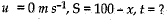Using the equation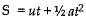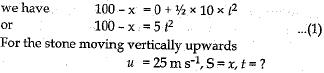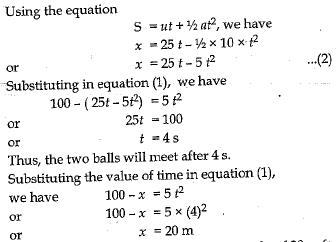Q.23. (a) What is the relation between the mass m and the weight w of a body?
(b) What are the differences between mass and weight?
Ans.
(a) Weight of a body is the force of attraction of the earth on that body. This force depends on the mass (m) of the body and the acceleration due to gravity (g).
F = m x a
F = m x g
W = m x g
The weight (W) of the body is directly proportional to the mass of the body,
(b) Difference between mass and weight: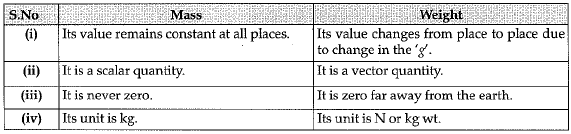Q.24. (i) How are pteriodophytes different from phanerogams?
(ii) Why do we classify organisms?

OR
Write any three differences between xylem and phloem.
Ans.
(i) Difference between pteriodophytes and phanerogams: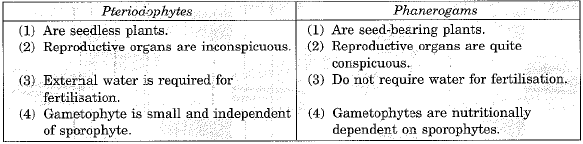(ii) The purpose of classifying organisms is to organise the vast number of known plants and animals into categories that could be identified, named, remembered and studied. Identification is not possible without any system of classification. It is not possible to study every organism. Study of few organisms gives a fair idea about other members of the group. Classification helps in bringing out similarities and dissimilarities amongst organisms. They indicate the evolutionary pathways. Moreover organisms of other regions as well as fossils can be studied only with the help of a system of classification.
OR
Differences between xylem and phloem :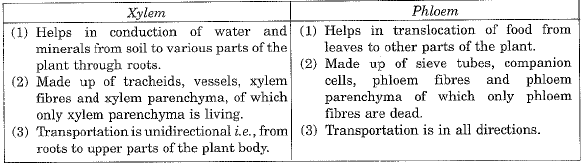SECTION - C

Q.25. (i) State Newton’s second law of motion.
(ii) Using second law of motion establish a mathematical formula correlating force with acceleration produced in an object due to it.
(iii) Define SI unit of force.
Ans. (i) Newton’s second law of motion states that the rate of change of momentum of an object is directly proportional to the external unbalanced force applied on it and takes place in the direction of applied force.
(ii) Let an object of mass m was initially moving with a velocity u. It means that its initial momentum P1 = mu.
If on applying a constant force F for time t the velocity of the object changes to v then final momentum of given object p2 = mv.
∴ Change in momentum = P2 - P1 = mv - mu = m(v - u)
∴ Rate of change of momentum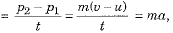where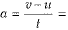acceleration of the given object.
According to statement of second law of motion, the rate of change of momentum is directly proportional to the applied force. Hence, we have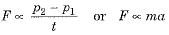⇒ F= kma, where k is a constant of proportionality.
Generally the units of force are so selected that if m = 1 and a = 1, then force F = 1. In such a case value of constant k becomes 1 and the relation becomes:
F = ma
(iii) The SI unit of force is a newton (1 N), which is the force which produces an acceleration of 1 ms-2 when applied on an object of mass 1 kg.

Q.26. In what way is the Rutherford's atomic model different from that of Thomson's atomic model?
Ans.
Rutherford proposed a model in which electrons revolve around the nucleus in well-defined orbits. There is a positively charged centre in an atom called the nucleus. He also proposed that the size of the nucleus is very small as compared to the size of the atom and nearly all the mass of an atom is centred in the nucleus. Whereas Thomson proposed the model of an atom to be similar to a Christmas pudding. The electrons are studded like currants in a positively charged sphere like Christmas pudding and the mass of the atom was supposed to be uniformly distributed.

Q.27. (a) A sample of vitamin C is known to contain 2.58 x 1024 oxygen atoms. How many moles of oxygen atoms are present in the sample?
(b) Write one word for the following:
(i) In a balanced chemical equation,, the sum of the masses of reactants and products remains unchanged.
(ii) A group of atoms carrying a fixed charge on them.
(c) Write chemical formulae of the following compounds:
(i) Sodium phosphate
(ii) Ammonium carbonate
OR
Verify by calculating that:
(i) 5 mole of CO2 and 5 mole of H2S do not have the same mass.
(ii) 240 gm of Ca and 240 gm of Mg elements have molar ratio 3 : 5.
(Atomic mass of C = 12 u, O = 16 u, H = 1 u, S = 32 u, Ca = 40 u, Mg = 24 u)
Ans.
(a) Number of moles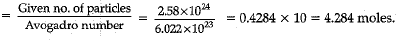(b) (i) Law of conservation of mass.
(ii) Ion
(c) (i) Na3PO4
(ii) (NH4)2CO3
OR
(i) Molecular mass of CO2 = 44 u
5 moles of CO2 = 5 x 44 = 220 gm
Molecular mass of H2S = 34 u
5 moles of H2S = 5 x 34 = 170 gm
Hence, both do not have same mass.
(ii) 40 gm of Ca = 1 mole
240 gm of Ca = 1/40 x 240 = 6 moles
24 gm of Mg = 1 mole
240 gm of Mg = 1/24 x 240 = 10 moles
∴ Molar ratio is 6 : 10 i.e., 3 : 5

Q.28. (a) Give two characteristics of the following phylum:
(i) Platyhelminthes
(ii) Arthropoda
(iii) Porifera.
(b) What is notochord and explain its function?

OR
(a) Name a vaccine which saves the life of babies from three diseases.
(b) How principle of immunisation is implemented for eliminating polio?
(c) Name two diseases which are caused in due course of time and last life long i.e., they can be kept in control but cannot be cured permanently.
(d) Why female anopheles mosquito feeds on human blood?
(e) Give the causative organism of Malaria.
Ans.
(a) (i) Platyhelminthes: A phylum of flatworms where the body is dorsoventrally flattened, triploblastic but acoelomate with organ level of organisation and bilateral symmetry. They possess soft, elongated leaf like or tape like body.
(ii) Arthropoda: A phylum of triploblastic, bilaterally symmetrical segmented animals having jointed legs and appendages a chitinous exoskeleton and a blood filled body cavity called haemocoel. It is the largest group of animals, which are found in variety of habitats like land, moist soil, fresh water, marine water as well as on the bodies of other animals.
(iii) Porifera: Porifera is a phylum of diploblastic, acoelomate animals having cellular level organisation, porous body with canal system and sedentary habit. The body has a covering of hard skeleton which comprises of spicules of calcium carbonate, silica or spongin fibres.
(b) A notochord is a solid rod present in chordates at some stage.
It provides place for muscles to attach. It increases internal support and locomotory power.
OR
(a) DPT is a triple vaccine which saves the life of babies from diphtheria, whooping cough (pertussis) and tetanus.
(b) Polio drops are heat killed or chemically weakened pathogens which are given periodically as booster doses to children under five years of age. These preparations stimulate the body to produce antibodies against these antigens. Thus, body becomes immune to the polio disease.
(c) High blood pressure and diabetes are two diseases which are caused in due course of time and last life long. They can be kept in control but cannot be cured permanently.
(d) Female anopheles mosquito feeds on human blood as it requires large amount of protein to lay their eggs.
(e) Malaria is caused by Plasmodium, also known as malarial parasite.

Q.29.  A stone is thrown in a vertically upward direction with a velocity of 5 m s-1. If the acceleration of the stone during its motion is 10 m s-2 in the downward direction, what will be the height attained by the stone and how much time will it take to reach there?
Ans.
Given:
Initial velocity, u = 5 m s-1
Final velocity, v = 0
Acceleration in the downward direction == 10 m s-2
Therefore, acceleration in the upward direction
a = - 10 m s-2
Height attained by stone, S = ?
Time taken to attain height, t = ?
(i) Using the relation; v = u + at
0 = 5 + (-10) t
Or
t =5/10 = 0.5 s
(ii) Using the relation; v2 - u2 = 2 aS, we have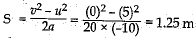Q.30. (a) State the criterion for the classification of organisms as belonging to kingdom Monera or Protista.
(b) State the kingdom in which you will place an organism which is single celled, eukaryotic and photosynthetic.
(c) List four conventions which are followed while writing scientific names of living organisms.
Ans.
(a) The criterion for classification of kingdom Monera and Protista is the type of cell they are made up of, Monera are prokaryotic organisms. They do not possess weE defined nucleus or organelles and also do not exhibit multicellular body design.
Protista are unicellular eukaryotic organisms having membrane bound cell organelles.
(b) Protista.
(c) Certain conventions are followed while writing the scientific names:
(i) The name of the genus begins with a capital letter.
(ii) The name of the species begins with a small letter.
(iii) When printed, the scientific name is given in italics.
(iv) When written by hand, the genus name and the species name have to be underlined separately.

Offer running on EduRev: Apply code STAYHOME200 to get INR 200 off on our premium plan EduRev Infinity!

135 docs

,

,

,

,

,

,

,

,

,

,

,

,

,

,

,

,

,

,

,

,

,

;